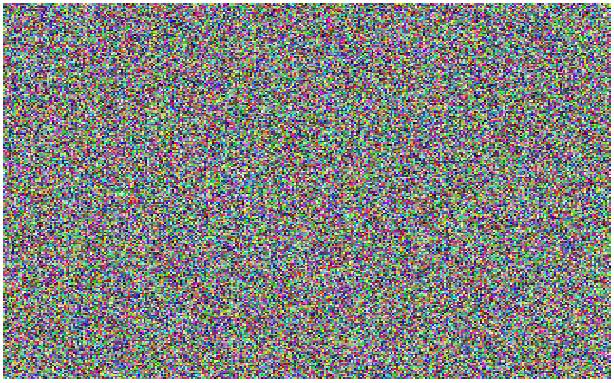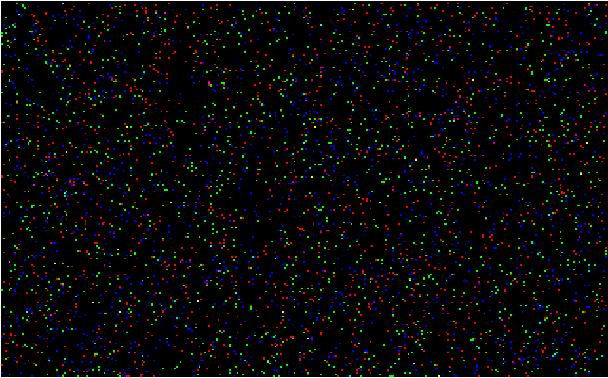Example 1.  Temporal Color Changes in a Stack of Images Containing Randomly Generated Colors

In the image (1.1) below, you see the first of four spatial (x, y) images generated as a function of time (t) by our software running in Mathematica.Image 1.1. Randomly colored pixels in image 1 of a stack of 4 images.

The pixels' colors are made by calling a random number generator to construct hundreds of thousands of RGB values.  The other three images in this image stack are generated similarly, thus each pixel's color changes in time.  This image stack is not very interesting until we start to visualize patterns that emerge from this randomness by a selection method employed below.  The spatial dimensions of this image stack are 320 x 240, with a total of four images in the time course.)Image 1.2.  Pixels are shown if their color, per each of four times, increases.

Image 1.2, shown above, is a pseudocolor representation of the temporal image stack after color-selection criteria are applied with respect to time.  These criteria select pixels to be colorized if and only if they show consistent color changes over all four time frames:  Pixels that become bluer with time are encoded as blue (B), those becoming greener with time are encoded as green (G), and pixels becoming redder with time are encoded as red (R).  For some pixels, coincident changes in two channels are observed; those pixels are pseudocolored in an additive manner:  R + B =  magenta (M), G + B = cyan (C), and G + R = yellow (Y).  In rare instances, all 3 values simultaneously increase in time, and those pixels are pseudocolored white (W = R + G + B).  Each time the program is run, a unique image is produced.

This technique is not limited to ordinary, three channel RGB images:  Pseudocoloring techniques can also be applied to hyperspectral data, wherein more than three spectroscopic channels are used in the initial data set.  All of these ideas are fundamentally consistent with the concept of the mathematical tensor.  As such, no physical embodiment of the mathematics needs to be envisioned.  Instead, we might, for example, consider the pseudocoloring that is described above to be simply a method of analyzing random noise in a rank three tensor.  Still another embodiment of this analysis would replace the concept of time with a third spatial axis, thus reducing a 3-dimensional color cube to two dimensions - wherein the pseudocoloring then represents a spatial trend in color change across the third dimension of the color cube.

Suggestions for further work include expanding the number of channels into hyperspectral (>3) channels, and increasing the number of images (time points).

Source code in .nb and .PDF formats.